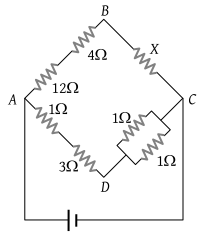# NEET Questions Solved

PMT - 1986

In the circuit shown in the adjoining figure, the current between B and D is zero, the unknown resistance is of(1) 4 Ω

(2) 2 Ω

(3) 3 Ω

(4) em.f. of a cell is required to find the value of X

(2) By balanced Wheatstone bridge condition $\frac{16}{X}=\frac{4}{0.5}$

$X=\frac{8}{4}=2\text{\hspace{0.17em}}\Omega$

Difficulty Level:

• 11%
• 62%
• 15%
• 13%